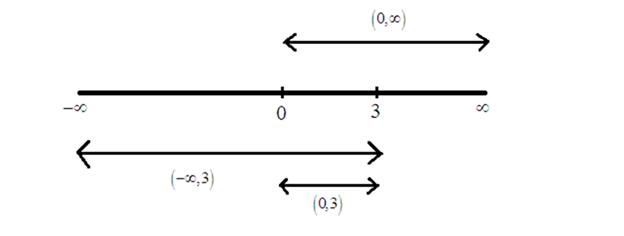# Explain that the method is correct or not.### Precalculus: Mathematics for Calcu...

6th Edition
Stewart + 5 others
Publisher: Cengage Learning
ISBN: 9780840068071### Precalculus: Mathematics for Calcu...

6th Edition
Stewart + 5 others
Publisher: Cengage Learning
ISBN: 9780840068071

#### Solutions

Chapter 1.7, Problem 123E
To determine

## Explain that the method is correct or not.

Expert Solution

Solution taken as (,3) is wrong.

### Explanation of Solution

Calculation:

Consider an inequality is 1<3x

Multiplying both sides by x .to get x<3 .

That is, 1<3x

x<3x  when x>0 x(,3)&x(0,)x(0,3)

Consider the line graphHence, 1<3xx<3 is possible only when x>0 .

Hence, the solution taken as (,3) is wrong.

### Have a homework question?

Subscribe to bartleby learn! Ask subject matter experts 30 homework questions each month. Plus, you’ll have access to millions of step-by-step textbook answers!